Next: Conclusions Up: Round Robin Classification Previous: Imbalanced Class Distributions:   Contents

Related Work

The idea of pairwise classification has been known in the neural networks and statistics communities for some time. For example, Witten and Frank (2000, p.113) refer to it as a technique for making linear regression applicable to multi-class problems but do not cite a source. The earliest reference we could find is Knerr et al. (1990) who propose a stepwise procedure for linearizing non-linear multi-class problems by first trying to identify classes that can be solved by a one-against-all approach, then a pairwise approach, and finally a piece-wise linear technique. The motivation behind this and other works in the neural networks community is that it is often better to have a modular network, i.e., a network that consists of several simpler and independently trained sub-networks, rather than a single, complex multi-layer neural network, which usually requires a large hidden layer and significant training times. Unordered (Anand et al., 1995) and pairwise (Lu and Ito, 1999) binarization techniques have also been investigated in this context.

Friedman (1996) evaluates pairwise classification on two versions of CART (Breiman et al., 1984) and a nearest neighbor algorithm on 50 randomly generated problems. He observed improvements for the CART version which uses a linear function for splitting a node and for the nearest neighbor rule. For CART with axis parallel splits, the performance of the pairwise class binarization was similar to that of the standard techniques. The author also provided a brief discussion of the complexity of the approach.

Naturally, pairwise classification was also investigated within the support vector machine community. A comparison of unordered and round robin binarization on a speaker identification problem can be found in (Schmidt, 1996; Schmidt and Gish, 1996), without providing a clear conclusion about the preferable approach. Kreßel (1999) discusses the technique in some detail and presents empirical results on a digit recognition task, where the author notes the unexpected efficiency of the approach without providing an explanation for it. Recently, Hsu and Lin (2002) conducted an excellent empirical comparison between unordered binarization, pairwise classification with both the simple voting technique we used and with the more efficient proposal for organizing the classifiers into an efficient DAG (Platt et al., 2000), as well as two approaches for directly generalizing the support vector algorithm to multi-class problems. In their experiments, round robin binarization performed best, both in terms of accuracy and training time. Interestingly, the advantage over competing methods was more pronounced for a linear kernel than for a non-linear RBF kernel. We interpret this as evidence that round robin binarization simplifies the individual binary decision problems as motivated in Figure 1.

Angulo and Català (2000) suggest a related technique where multi-class problems are mapped to 3-class problems. Like with pairwise classification, the idea is to generate one training set for each pair of classes, but in addition to encoding the two class values with target values +1 and -1, examples of all other classes are added with a target value of 0, which gives up some of the advantages that result from the reduction of the training set sizes on the binary problems. They do not evaluate their approach. A similar idea was used by Kalousis and Theoharis (1999) for the meta-learning task of predicting the most suitable learning algorithm(s) for a given dataset. A nearest-neighbor learner was trained to predict the better algorithm for each pair of learning algorithms, where each of these pairwise problems had three classes: one for each algorithm and a third class tie'' if both algorithms performed indistinguishably.

Error-correcting output codes (Dietterich and Bakiri, 1995) are a popular and powerful class binarization technique. The basic idea is to encode a c-class problem as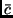binary problems (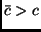), where each binary problem uses a subset of the classes as the positive class and the remaining classes as a negative class. It may thus be viewed as a generalization of unordered binarization, where only single classes are used as positive examples. As a consequence, each original class is encoded as a-dimensional binary vector, one dimension for each prediction of a binary problem (by convention +1 for positive and -1 for negative). The resulting matrix of the form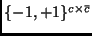is called the coding matrix. New examples are classified by determining the row in the matrix that is closest to the binary vector obtained by submitting the example to theclassifiers. If the binary problems are chosen in a way that maximizes the distance between the class vectors, the reliability of the classification can be significantly increased. Error-correcting output codes can also be easily parallelized, but each subtask requires the total training set. As typically, the penalty function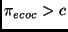, i.e., pairwise and unordered binarization are more efficient.

Allwein et al. (2000) show that a generalization of error-correcting output codes can be used as a general framework for class binarization techniques. Their basic idea is to generalize the coding matrices in a way that allows examples to be ignored in the binary problems, i.e., to allow the coding matrices to be of the form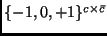. Clearly, pairwise classification is a special case in this framework. For example, the coding matrix for a double round robin has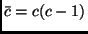columns, where the column corresponding to the binary problem <i,j> has +1 in the i-th row, -1 in the j-th row and 0 in all other rows. Thus, each row is a vector of the form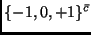. Note, however, that the vector of predictions is of the form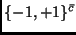because every binary classifier will make a prediction (either +1 or -1) for every example. Nevertheless, the simple voting procedure we used is equivalent to finding the row that is most similar to the prediction vector (if similarity is measured with the Hamming distance), which is equivalent to the decoding technique suggested by Dietterich and Bakiri (1995). Allwein et al. (2000) criticize this simple Hamming decoding and suggest the use of loss-based decoding techniques that take into account the confidence of the base learner into its own predictions. In a theoretical analysis, which relates the training error of decoding methods to the error on the binary problems and to the minimum distance between entries in the coding matrix, the authors derive upper bounds for the training error of loss-based decoding that are lower than those for Hamming decoding. In an experimental study with five different class binarization techniques and three decoding techniques (two of them loss-based and one Hamming decoding), the loss-based techniques seemed to produce lower generalization errors. Their results also showed that for support vector machines unordered binarization is inferior to all other techniques, among them pairwise classification. Among these alternatives, no clear winner emerged. However, for boosted decision trees, unordered binarization performed on the same level as all other approaches.

Finally, we note the relation of round robin classification to comparison training (Utgoff and Clouse, 1991; Tesauro, 1989), which has been proposed as a training procedure in evaluation function learning. In this framework, the learner is not trained with the target values of the evaluation function in certain states, but instead is trained on state pairs where the preferable state is marked. Thus, this training procedure is somewhere between supervised learning (where the function is trained on examples of target values) and reinforcement learning (where it only receives indirect feedback about the value of states). Tesauro (1989) demonstrated a particularly interesting technique, where he enforced a symmetric neural network architecture and showed that with this architecture, one only has to perform n network evaluations to determine the best of n moves. It is an interesting open question, whether a similar technique could be employed for speeding up pairwise classification.Next: Conclusions Up: Round Robin Classification Previous: Imbalanced Class Distributions:   Contents
Johannes Fürnkranz 2002-03-11